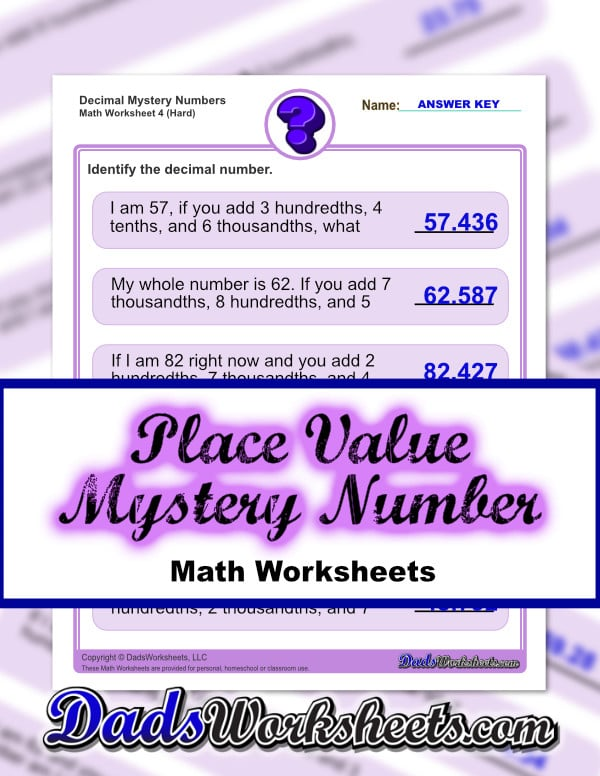# Place Value Worksheets

Place value is a fundamental concept that allows us to express arbitrarily large or small values using our base 10 numbering system. The place value worksheets on gradually build skills with identifying the place values in whole and decimal numbers, and learning how to perform basic place value arithmetic.

Expanded form is a concept directly related to place value, and the worksheets below provide practice problems converting numbers between expanded form (sometimes called place value form), standard form ("normal" numbers) and word form.

You'll also find a link to a set of place value charts that students can use to break down numbers by digit into place values and periods.

## Decimal Place Value Mystery Numbers

### 12 Place Value Worksheets

These mystery number worksheets have students form decimal numbers by combining descriptions of place values in word form.

Decimal Place Value

## Standard, Expanded and Word Form

### 170 Place Value Worksheets

Practice worksheets for converting numbers between standard form (digits), expanded form (place value) and word form (spelled out or spoken representation).

Standard, Expanded, Word Form

### 20 Addition Place Value Worksheets

These place value worksheets for addition are good first steps towards multi-digit addition problems. These problems require students to add multi-digit values without combining anything in each place value column.

## Place Value Subtraction

### 8 Subtraction Place Value Worksheets

Place value worksheets for subtraction. These primary worksheets are a good lead into the multiple digit subtraction worksheets, but focus on subtracting digits without interaction between different place values in the problem.

Place Value Subtraction

## Place Value Chart

When the numbers get longer, place value charts can help teach students how to read numbers and differentiate between smaller and larger values even when decimals get involved.

Place Value Chart